Refer to our Texas Go Math Grade 5 Answer Key Pdf to score good marks in the exams. Test yourself by practicing the problems from Texas Go Math Grade 5 Unit 4 Assessment Answer Key.

Vocabulary

Choose the best term

Question 1.
A ___ is a polygon with nine sides and nine angles, (p, 409)
Answer: A nonagon is a polygon with nine sides and nine angles.

Question 2.
The vertical number line on a coordinate grid is the ___. (p. 511)
Answer: The vertical number line on a coordinate grid is the y-axis.

Concepts and Skills

Convert. TEKS 5.7.A

Question 3.
5kg = ___ g
Convert from kilograms to grams
1 kg = 1000 grams
5 kg = 5 × 1000 grams = 5000 grams

Question 4.
65 yd 2 ft = ___ ft
Convert from yards to feet.
1 yard = 3 feet
65 yard = 195 feet
195 feet 2 feet = 197 feet

Question 5.
500 min = __ hr __ min
Convert from minutes to hours.
60 min = 1 hour
500 mins = 8 hour 33 minutes

Classify each figure in as many ways as possible. TEKS 5.5.A

Question 6.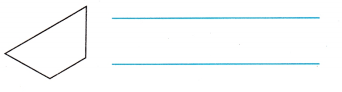Trapezoid

Question 7.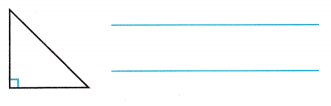Right triangle
triangle

Use the table for 8-9. TEKS 5.8.C

Question 8.
The rule for the pattern is y = x + 4. Complete the table and write related number pairs of data as ordered pairs.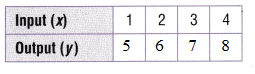Question 9.
Graph the data in a coordinate grid.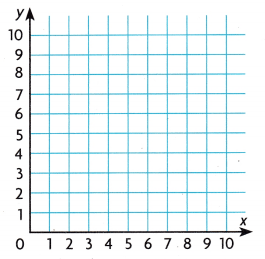Texas Test Prep

Question 10.
Sam needs to order a container that can hold 5,200 milliliters of molasses. Which of the following containers is NOT large enough to hold the molasses? TEKS 5.7.A
(A) a 10-liter barrel
(B) a 5-liter barrel
(C) a 50-liter barrel
(D) a 6-liter barrel

Explanation:
Sam needs to order a container that can hold 5,200 milliliters of molasses
5000 milliliters = 5 liters
So, from the above options 5 liter barrel cannot hold 5200 milliliters of molasses.
Thus the answer is option B.

Question 11.
A shipping container is 20 meters long, 10 meters wide, and 8 meters high. What is the greatest number of cartons that can fit in the container if each carton has a volume of 1 cubic meter? TEKS 5.6.A
(A) Not here
(B) 16,000
(C) 1,600
(D) 2,000

Explanation:
A shipping container is 20 meters long, 10 meters wide, and 8 meters high.
V = lwh
V = 20 × 10 × 8
V = 1600 cu. meter
Thus the correct answer is option C.

Question 12.
Which of the following can be used for the unknown shape in the graphic organizer? TEKS 5.5.A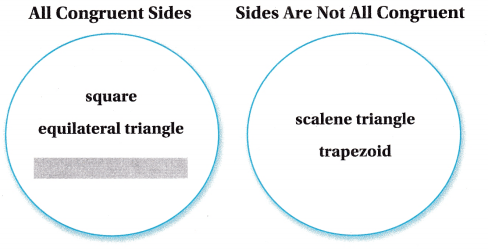(A) isosceles triangle
(B) rhombus
(C) right triangle
(D) irregular pentagon

Question 13.
Leah uses the rule y = 8x to determine the number of legs spiders have. If the number of spiders is the x-coordinate and the total number of legs is the y- coordinate, which ordered Iair can Leah use to show the
number of legs for 8 spiders? TEKS 5.8.C(A) (8, 8)
(B) (64, 8)
(C) (8, 60)
(D) (8, 64)

Question 14.
Delia has a 2 gallon container filled with fruit punch. How many 8 fluid ounce glasses of punch can she serve from her container? TEKS 5.7.A
(A) 22
(B) 32
(C) 16
(D) 44

Question 15.
A large wooden crate is used to store glass blocks used for making special glass walls. Each block is a one-inch cube. If 256 blocks fit in the bottom laver of the crate and the crate is 12 inches tall, what is the volume of the wooden crate? TEKS 5.6.B
(A) 3,072 cubic inches
(B) 192 cubic inches
(C) 64 cubic inches
(D) 768 cubic inches
A large wooden crate is used to store glass blocks used for making special glass walls.
Each block is a one-inch cube.
256 blocks fit in the bottom laver of the crate and the crate is 12 inches tall.
256 × 12 = 3072 cubic inches
Thus the correct answer is option A.

Question 16.
Mayang has 2 pounds of beef. She uses 18 ounces to make a stew. How much more beef should she buy if she needs 2 pounds to make burgers? TEKS 5.7.A
(A) 1 lb
(B) 16 oz
(C) 1 lb 2 oz
(D) 1 lb 14 oz

Question 17.
Amy’s design features a polygon with congruent sides. All of the angles are obtuse. Which of the following polygons could be in Amy’s design? TEKS 5.5.A
(A) equilateral triangle
(B) square
(C) regular pentagon
(D) trapezoid

Question 18.
The points on the coordinate grid represent the locations of the first four holes on a golf course.
Which ordered pair describes the Location of the hole labeled T? TEKS 5.8.C
(A) (0, 7)
(B) (1, 7)
(C) (7, 0)
(D) (7, 1)Question 19.
Raul builds these models using centimeter cubes. What is the difference in volume between the two models? TEKS 5.6.A(A) 48 cubic centimeters
(B) 2 cubic centimeters
(C) 3 cubic centimeters
(D) 140 cubic centimeters
The volume of each cube is 1
The Sum of 7 cubes is 7
The Sum of 5 cubes is 5
7 – 5 = 2
Thus the correct answer is option B.

Question 20.
A scientist is measuring the length of insects. One kind of insect is 1.25 decimeters long. What is its length in millimeters? TEKS 5.7.A
(A) 0.0125 mm
(B) 12.5 mm
(C) 125 mm
(D) 0.125 mm

Question 21.
Point Wis 5 units to the right and 3 units up from the origin. What ordered pair describes point W? TEKS 5.8.A, 5.8.B
(A) (5, 0)
(B) (5, 3)
(C) (3, 5)
(D) (0, 5)

Question 22.
Parker makes 5 liters of iced tea for a party. He pours the tea into smaller bottles for the guests. If each bottle contains 250 milliliters, how many bottles can Parker fill? TEKS 5.7.A
(A) 5
(B) 20
(C) 10
(D) 4